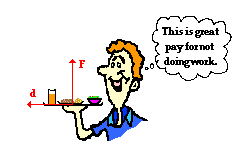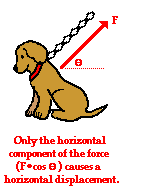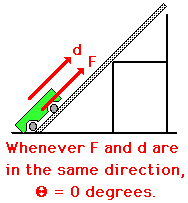Work, Energy, and Power - Lesson 1 - Basic Terminology and Concepts

# Definition and Mathematics of Work

In the first three units of The Physics Classroom, we utilized Newton's laws to analyze the motion of objects. Force and mass information were used to determine the acceleration of an object. Acceleration information was subsequently used to determine information about the velocity or displacement of an object after a given periodof time. In this manner, Newton's laws serve as a useful model for analyzing motion and making predictions about the final state of an object's motion. In this unit, an entirely different model will be used to analyze the motion of objects. Motion will be approached from the perspective of work and energy. The effect that work has upon the energy of an object (or system of objects) will be investigated; the resulting velocity and/or height of the object can then be predicted from energy information. In order to understand this work-energy approach to the analysis of motion, it is important to first have a solid understanding of a few basic terms. Thus, Lesson 1 of this unit will focus on the definitions and meanings of such terms as work, mechanical energy, potential energy, kinetic energy, and power.

When a force acts upon an object to cause a displacement of the object, it is said that work was done upon the object. There are three key ingredients to work - force, displacement, and cause. In order for a force to qualify as having done work on an object, there must be a displacement and the force must cause the displacement. There are several good examples of work that can be observed in everyday life - a horse pulling a plow through the field, a father pushing a grocery cart down the aisle of a grocery store, a freshman lifting a backpack full of books upon her shoulder, a weightlifter lifting a barbell above his head, an Olympian launching the shot-put, etc. In each case described here there is a force exerted upon an object to cause that object to be displaced.Read the following five statements and determine whether or not they represent examples of work. Then click on the See Answer button to view the answer.

 Statement Answer with Explanation A teacher applies a force to a wall and becomes exhausted. See AnswerNo. This is not an example of work. The wall is not displaced. A force must cause a displacement in order for work to be done. A book falls off a table and free falls to the ground. See AnswerYes. This is an example of work. There is a force (gravity) which acts on the book which causes it to be displaced in a downward direction (i.e., "fall"). A waiter carries a tray full of meals above his head by one arm straight across the room at constant speed. (Careful! This is a very difficult question that will be discussed in more detail later.) See AnswerNo. This is not an example of work. There is a force (the waiter pushes up on the tray) and there is a displacement (the tray is moved horizontally across the room). Yet the force does not cause the displacement. To cause a displacement, there must be a component of force in the direction of the displacement. A rocket accelerates through space. See AnswerYes. This is an example of work. There is a force (the expelled gases push on the rocket) which causes the rocket to be displaced through space.

### Work Equation

Mathematically, work can be expressed by the following equation.

W = F • d • cos Θ

where F is the force, d is the displacement, and the angle (theta) is defined as the angle between the force and the displacement vector. Perhaps the most difficult aspect of the above equation is the angle "theta." The angle is not just any 'ole angle, but rather a very specific angle. The angle measure is defined as the angle between the force and the displacement. To gather an idea of it's meaning, consider the following three scenarios.• Scenario A: A force acts rightward upon an object as it is displaced rightward. In such an instance, the force vector and the displacement vector are in the same direction. Thus, the angle between F and d is 0 degrees.

• Scenario B: A force acts leftward upon an object that is displaced rightward. In such an instance, the force vector and the displacement vector are in the opposite direction. Thus, the angle between F and d is 180 degrees.

• Scenario C: A force acts upward on an object as it is displaced rightward. In such an instance, the force vector and the displacement vector are at right angles to each other. Thus, the angle between F and d is 90 degrees.

### To Do Work, Forces Must Cause DisplacementsLet's consider Scenario C above in more detail. Scenario C involves a situation similar to the waiter who carried a tray full of meals above his head by one arm straight across the room at constant speed. It was mentioned earlier that the waiter does not do work upon the tray as he carries it across the room. The force supplied by the waiter on the tray is an upward force and the displacement of the tray is a horizontal displacement. As such, the angle between the force and the displacement is 90 degrees. If the work done by the waiter on the tray were to be calculated, then the results would be 0. Regardless of the magnitude of the force and displacement, F*d*cosine 90 degrees is 0 (since the cosine of 90 degrees is 0). A vertical force can never cause a horizontal displacement; thus, a vertical force does not do work on a horizontally displaced object!!

It can be accurately noted that the waiter's hand did push forward on the tray for a brief period of time to accelerate it from rest to a final walking speed. But once up to speed, the tray will stay in its straight-line motion at a constant speed without a forward force. And if the only force exerted upon the tray during the constant speed stage of its motion is upward, then no work is done upon the tray. Again, a vertical force does not do work on a horizontally displaced object.The equation for work lists three variables - each variable is associated with one of the three key words mentioned in the definition of work (force, displacement, and cause). The angle theta in the equation is associated with the amount of force that causes a displacement. As mentioned in a previous unit, when a force is exerted on an object at an angle to the horizontal, only a part of the force contributes to (or causes) a horizontal displacement. Let's consider the force of a chain pulling upwards and rightwards upon Fido in order to drag Fido to the right. It is only the horizontal component of the tension force in the chain that causes Fido to be displaced to the right. The horizontal component is found by multiplying the force F by the cosine of the angle between F and d. In this sense, the cosine theta in the work equation relates to the cause factor - it selects the portion of the force that actually causes a displacement.

### The Meaning of ThetaWhen determining the measure of the angle in the work equation, it is important to recognize that the angle has a precise definition - it is the angle between the force and the displacement vector. Be sure to avoid mindlessly using any 'ole angle in the equation. A common physics lab involves applying a force to displace a cart up a ramp to the top of a chair or box. A force is applied to a cart to displace it up the incline at constant speed. Several incline angles are typically used; yet, the force is always applied parallel to the incline. The displacement of the cart is also parallel to the incline. Since F and d are in the same direction, the angle theta in the work equation is 0 degrees. Nevertheless, most students experienced the strong temptation to measure the angle of incline and use it in the equation. Don't forget: the angle in the equation is not just any 'ole angle. It is defined as the angle between the force and the displacement vector.### The Meaning of Negative Work

On occasion, a force acts upon a moving object to hinder a displacement. Examples might include a car skidding to a stop on a roadway surface or a baseball runner sliding to a stop on the infield dirt. In such instances, the force acts in the direction opposite the objects motion in order to slow it down. The force doesn't cause the displacement but rather hinders it. These situations involve what is commonly called negative work. The negative of negative work refers to the numerical value that results when values of F, d and theta are substituted into the work equation. Since the force vector is directly opposite the displacement vector, theta is 180 degrees. The cosine(180 degrees) is -1 and so a negative value results for the amount of work done upon the object. Negative work will become important (and more meaningful) in Lesson 2 as we begin to discuss the relationship between work and energy.

### Units of Work

Whenever a new quantity is introduced in physics, the standard metric units associated with that quantity are discussed. In the case of work (and also energy), the standard metric unit is the Joule (abbreviated J). One Joule is equivalent to one Newton of force causing a displacement of one meter. In other words,

The Joule is the unit of work.

1 Joule = 1 Newton * 1 meter

1 J = 1 N * m

In fact, any unit of force times any unit of displacement is equivalent to a unit of work. Some nonstandard units for work are shown below. Notice that when analyzed, each set of units is equivalent to a force unit times a displacement unit.

### Non-standard Units of Work:

 foot•pound kg•(m/s2)•m kg•(m2/s2)

In summary, work is done when a force acts upon an object to cause a displacement. Three quantities must be known in order to calculate the amount of work. Those three quantities are force, displacement and the angle between the force and the displacement.

### Investigate!

We do work every day. The work we do consumes Calories ... err, should we say Joules. But how much Joules (or Calories) would be consumed by various activities? Use the Daily Work widget to investigate the amount of work that would be done to run, walk or bike a for a given amount of time at a specified pace.

Click to continue the lesson on Work

### We Would Like to Suggest ...Sometimes it isn't enough to just read about it. You have to interact with it! And that's exactly what you do when you use one of The Physics Classroom's Interactives. We would like to suggest that you combine the reading of this page with the use of our It's All Uphill Interactive. You can find it in the Physics Interactives section of our website. The It's All Uphill Interactive allows a learner to explore the effect of an incline angle upon the force and the work done in pulling a cart up a hill at a constant speed.

Next Section:
Jump To Next Lesson:

Follow Us